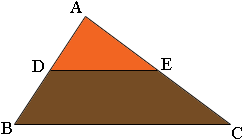SEARCH HOMEMath Central Quandaries & QueriesQuestion from Nazrul, a teacher: ABC is a triangle , D is the mid point of AB and DE is parallel to BC where E lies on AC. How can I prove that E is the mid point of AC. Thank you for answering my previous question.Nazrul,DE is parallel to BC. What does that tell you about angles ADE and ABC? What does that tell you about triangle ADE and ABC?

PennyMath Central is supported by the University of Regina and The Pacific Institute for the Mathematical Sciences.